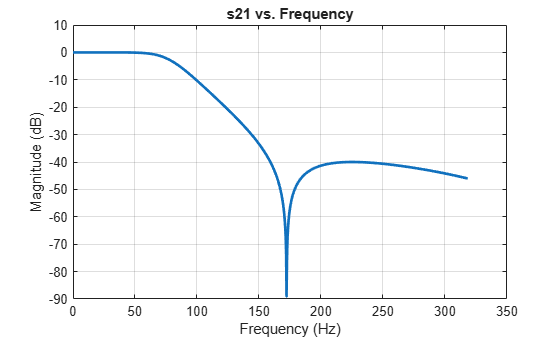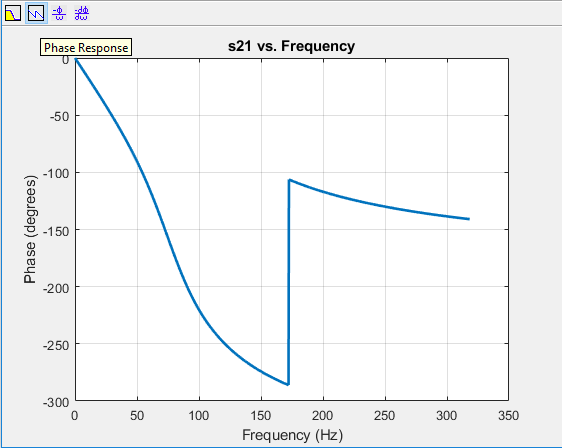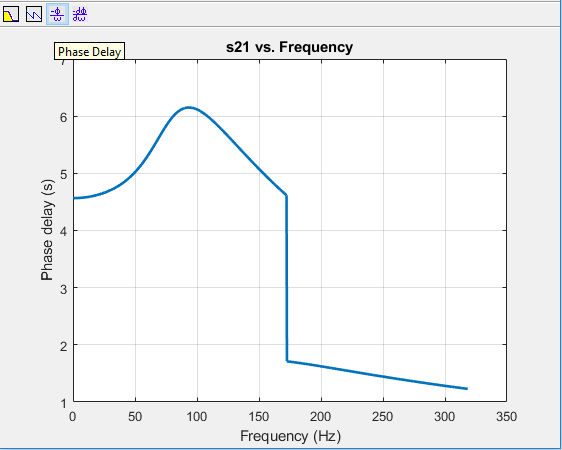Design, Visualize and Explore Inverse Chebyshev Filter - II

This example shows how to design a fourth-order inverse Chebyshev low-pass filter with stopband frequency of 10000 rad/sec, and epsilon of 0.01 (please see the reference section) using rffilter. This rffilter could be used in a circuit or in a rfbudget object.

The rffilter object is used to design a RF filter. A filter requires a minimum set for parameters to completely define it.

The parameters to design an inverse Chebyshev filter can be one of the following:

• Filter order, Passband frequency, Passband and Stopband Attenuation

• Passband and Stopband frequencies, Passband and Stopband Attenuation

• Filter order, Stopband frequency, Stopband Attenuation

Design Filter

N           = 4;                                    % Filter order
Fs          = 1000/(2*pi);                          % Stopband frequency
epsilon     = 0.01;
Rs          = 10*log10((1+epsilon^2)/epsilon^2);    % Stopband attenuation

Use the first set of parameters to define the filter.

r = rffilter('FilterType','InverseChebyshev','ResponseType','Lowpass',  ...
'Implementation','Transfer function','FilterOrder',N,               ...
'PassbandFrequency',Fs,'PassbandAttenuation',Rs,                    ...
'StopbandAttenuation',Rs);

Note: Alternative, you can also use the third set of parameters to design the same filter:

r = rffilter('FilterType','InverseChebyshev','ResponseType','Lowpass',  ...
'Implementation','Transfer function','FilterOrder',N,                   ...
'StopbandFrequency',Fs,'StopbandAttenuation',Rs);

The limitation of this parameter set is that it assumes the passband attenuation to be fixed at 10*log10(2) dB.

Visualize magnitude response, phase response, and phase delay of filter

frequencies = linspace(0,2*Fs,1001);
rfplot(r, frequencies);Optionally, you can also use Signal Processing Toolbox to visualize the analog filter using:

freqs(numerator{2,1},denominator)

Find zeros, poles, and gain

[z,p,k] = zpk(r);

You can obtain zeros, poles, and gain of Transfer function (S21) by:

format long g
zeros_21 = z{2,1}
zeros_21 = 4×1 complex

0.0000 + 1.0824i
0.0000 - 1.0824i
0.0000 + 2.6131i
0.0000 - 2.6131i

poles_21 = p                % Same denominator for S11, S12, S21 and S22
poles_21 = 4×1 complex

-1.7116 + 4.7610i
-1.7116 - 4.7610i
-5.0453 + 2.4079i
-5.0453 - 2.4079i

k_21     = k{2,1}
k_21 =
0.00999950003749688

View transfer function in factorized form

View these factor forms directly from the filter r.

disp('Numerator of Transfer function as factors:');
Numerator of Transfer function as factors:
r.DesignData.Numerator21
ans = 2×3

0.0000         0    1.1716
0.0000         0    0.0683

disp('Denominator of Transfer function as factors:');
Denominator of Transfer function as factors:
r.DesignData.Denominator
ans = 2×3

0.0000    0.0034    2.5596
0.0000    0.0101    3.1253

Alternatively, use |zpk| from Control System Toolbox to view the transfer function in factorized form.

G_s = zpk(zeros_21,poles_21,k_21)
G_s =

0.0099995 (s^2 + 1.172e06) (s^2 + 6.828e06)
-------------------------------------------------
(s^2 + 1009s + 3.125e05) (s^2 + 342.3s + 2.56e05)

Continuous-time zero/pole/gain model.

 Paarmann, L. D. Design and Analysis of Analog Filters: A Signal Processing Perspective. SECS 617. Boston: Kluwer Academic Publishers, 2001.